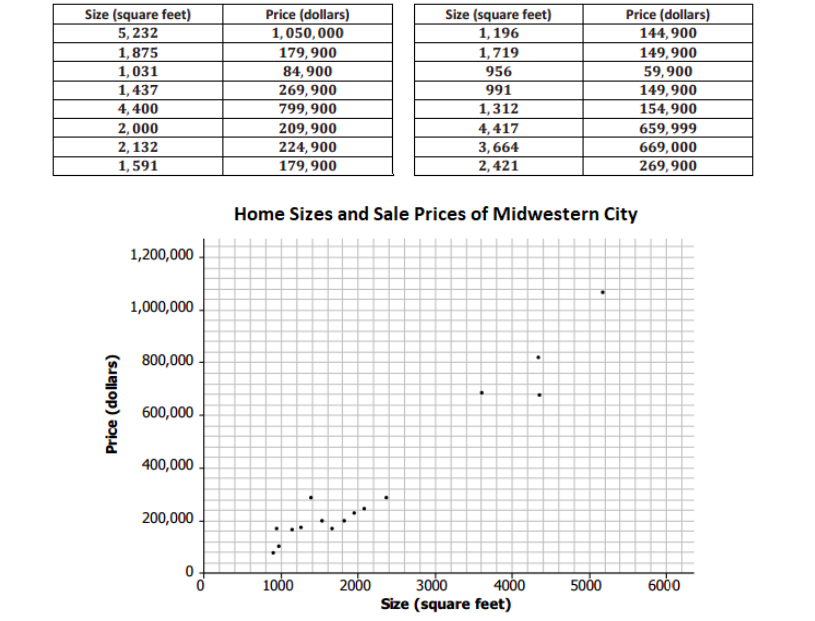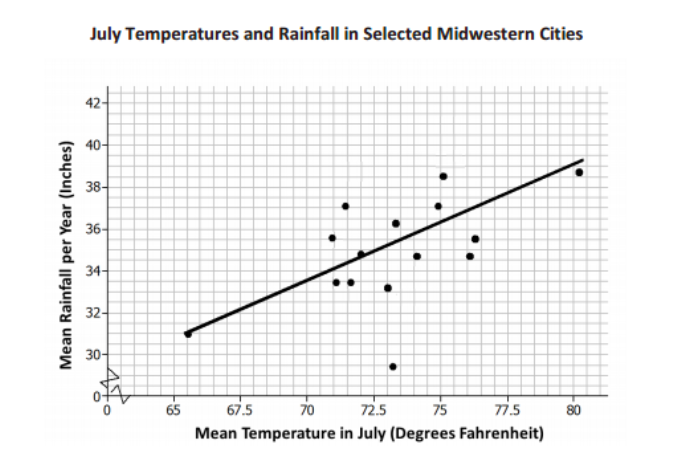# Bivariate Data

## Objective

Informally fit a line to data. Judge the fit of the line and make predictions about the data based on the line.

## Common Core Standards

### Core Standards

?

• 8.SP.A.2 — Know that straight lines are widely used to model relationships between two quantitative variables. For scatter plots that suggest a linear association, informally fit a straight line, and informally assess the model fit by judging the closeness of the data points to the line.

?

• 8.F.A.3

• 8.F.B.4

## Criteria for Success

?

1. Understand that a line can be drawn through a set of data in a scatter plot to represent a linear trend in the data.
2. Understand that a best fit line does not go through all or specific data points but rather is as close to as many data points as possible in a scatter plot; there is not one unique line that best fits a data set.
3. Draw a line through a data set to best represent a trend in the data.
4. Use a best fit line to make predictions about the data.

## Tips for Teachers

?

• In this lesson, students apply their understanding of linear relationships to scatter plots that have linear trends in the data. They realize that by drawing a line to best represent the trend in the data, they can better understand the relationship between the quantities and even make predictions (MP.4). In the next lesson, students write equations for these lines allowing students to analyze the relationships even more.
• Manipulatives that represent a line that can be maneuvered on paper are a valuable tool for this lesson; for example, wikisticks, raw spaghetti, thin pencils, etc.

#### Fishtank Plus

• Problem Set
• Student Handout Editor
• Vocabulary Package

## Anchor Problems

?

### Problem 1

For each graph shown below, draw a line that best represents the data points in the graph. Describe your thought process in drawing your lines.### Problem 2

The table below includes data from a Midwestern city about the sizes and sale prices of various homes sold in this city in 2013. The scatter plot that represents the table is also shown below.1. Describe the relationship you see between the two variables in the scatter plot.
2. Do you see any clusters or outliers? Explain.
3. Draw a line to best represent the trend in the data. Explain your strategy for determining where to draw the line.
4. Use your line to estimate the cost of a house in 2013 that had 3,000 square feet.

#### References

EngageNY Mathematics Grade 8 Mathematics > Module 6 > Topic B > Lesson 8Example 1

Grade 8 Mathematics > Module 6 > Topic B > Lesson 8 of the New York State Common Core Mathematics Curriculum from EngageNY and Great Minds. © 2015 Great Minds. Licensed by EngageNY of the New York State Education Department under the CC BY-NC-SA 3.0 US license. Accessed Dec. 2, 2016, 5:15 p.m..

Modified by The Match Foundation, Inc.

### Problem 3

This scatter diagram shows the lengths and widths of the eggs of some American birds.1. What does the graph show about the relationship between the lengths of birds' eggs and their widths?
2. Another sample of eggs from similar birds has an average length of 35 millimeters. If these bird eggs follow the trend in the scatter plot, about what width would you expect these eggs to have, on average?
3. Draw a line that best represents the trend in the data.
4. Explain why, in this case, it is not a good strategy to draw a line of best fit using point E (the farthest point to the right) and point (13, 9) (the farthest point to the left).

#### References

MARS Summative Assessment Tasks for Middle School Birds' Eggs

Birds' Eggs from the Summative Assessment Tasks for Middle School is made available through the Mathematics Assessment Project under the CC BY-NC-ND 3.0 license. Copyright © 2007-2015 Mathematics Assessment Resource Service, University of Nottingham. Accessed June 21, 2017, 11:01 a.m..

Modified by The Match Foundation, Inc.

## Problem Set

?The following resources include problems and activities aligned to the objective of the lesson that can be used to create your own problem set.

?

The plot below is a scatter plot of mean temperatures in July and mean inches of rain per year for a sample of Midwestern cities. A line is drawn to fit the data.1. Choose a point in the scatter plot and explain what it represents.
2. Use the line provided to predict the mean number of inches of rain per year for a city that has a mean temperature of 70°F in July.
3. Do you think the line provided is a good one for this scatter plot? Explain why or why not.
4. A fellow classmate uses the strategy of drawing a line so that half of the points are above the line and half of the points are below the line. Explain or show how this could result in a line that was not representative of the data.

#### References

EngageNY Mathematics Grade 8 Mathematics > Module 6 > Topic B > Lesson 8Exit Ticket

Grade 8 Mathematics > Module 6 > Topic B > Lesson 8 of the New York State Common Core Mathematics Curriculum from EngageNY and Great Minds. © 2015 Great Minds. Licensed by EngageNY of the New York State Education Department under the CC BY-NC-SA 3.0 US license. Accessed Dec. 2, 2016, 5:15 p.m..

Modified by The Match Foundation, Inc.

?